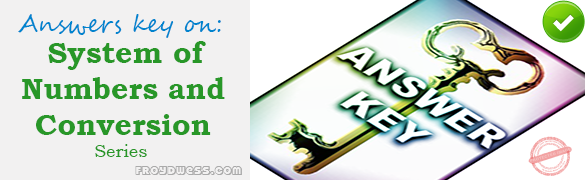# MCQ in System of Numbers and Conversion Part 3 – Answers

(Last Updated On: February 5, 2020)Below are the answers key for the Multiple Choice Questions in System of Numbers and Conversion Part 3.

101. C. 5

102. C. 345.96°

103. B. 24 cis 150°

104. C. 3 cis 30°

105. A. 5

106. B. 18 + i

107. B. 1 + 2i

108. A. i

109. D. 0

110. D. –46 + 9i

111. D. 5Լ143.13°

112. C. –1 + i

113. B. –√70

114. C. –21i

115. B. –8 + 4i

116. B. 5ei36.87°

117. D. 5 + 12i

118. B. ±3i

119. A. 2.565 + 1.176i

120. B. (1, -2)

121. B. √21

122. B. 6.00

123. C. Transitive Property

124. B. Symmetric Property

125. A. An axiom

126. C. extremes

127. D. lesser

128. C. Sample

129. D. Dispersion

130. C. Argand Diagram

131. B. Fermat’s series

132. B. Pyramidal number

133. A. Ogive

134. D. Divergent series

135. C. Rational

136. B. Determinant

137. A. theorem

138. C. like terms

139. B. Harmonic progression

140. A. Naperian logarithm

141. B. Constant variation

142. A. Zero

143. A. Solution set

144. C. an event

145. A. Trivial solutions

146. C. Phasor

147. C. Mode

148. C. Indeterminate

149. C. Zero

150. A. Arithmetic mean

151. A. Redundant equation

152. A. inequality

153. A. Function

154. D. Irrational equation

155. B. Probability

156. A. One is merely the negative of the other

157. C. It has no other integer as factor excepts itself or 1

158. B. an identity

159. A. a conditional equation

160. B. zero

### Online Questions and Answers in System of Numbers and Conversion Series

Following is the list of practice exam test questions in this brand new series:

System of Numbers and Conversion MCQs
PART 1: MCQs from Number 1 – 50                        Answer key: PART I
PART 2: MCQs from Number 51 – 100                      Answer key: PART II
PART 3: MCQs from Number 101 – 150                      Answer key: PART III